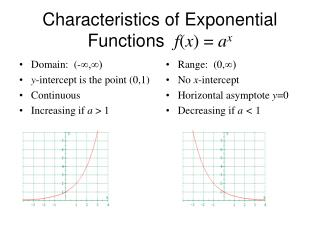DownloadDownload PresentationCharacteristics of Exponential Functions f ( x ) = a x

# Characteristics of Exponential Functions f ( x ) = a x

Download Presentation## Characteristics of Exponential Functions f ( x ) = a x

- - - - - - - - - - - - - - - - - - - - - - - - - - - E N D - - - - - - - - - - - - - - - - - - - - - - - - - - -
##### Presentation Transcript

1. Domain: (-∞,∞) y-intercept is the point (0,1) Continuous Increasing if a > 1 Range: (0,∞) No x-intercept Horizontal asymptote y=0 Decreasing if a < 1 Characteristics of Exponential Functions f(x) = ax